# How to Use Gaussian Elimination

Samuel Hoyland, Yuanxin (Amy) Yang Alcocer
• Author
Samuel Hoyland

I have a bachelor's in math and music from Wesleyan University. I am currently pursuing a doctorate of music in composition at the University of Georgia. I have 9 years of work experience writing math content and 7 years of experience tutoring math one-on-one.

• Instructor
Yuanxin (Amy) Yang Alcocer

Amy has a master's degree in secondary education and has been teaching math for over 9 years. Amy has worked with students at all levels from those with special needs to those that are gifted.

Learn about Gaussian elimination, one of the methods of solving a system of linear equations. Understand how to do Gaussian elimination with the help of an example. Updated: 10/17/2021

Show

## Systems of Linear Equations

A linear equation is an equation of degree 1 or less. In other words, linear equations are made up of one or more terms that are added or subtracted together and all of the terms must be composed of a number (called a coefficient, which can be 1) multiplied by at most one variable with no exponent. Some examples of such terms are {eq}7x {/eq}, {eq}\tfrac{2}{3}y {/eq}, {eq}a {/eq}, and {eq}5 {/eq}.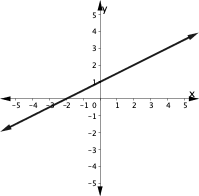The following are examples of linear equations.

• {eq}7x = 0 {/eq}
• {eq}\tfrac{2}{3}y - a = 9n {/eq}
• {eq}4t + 9 = t - \pi {/eq}

The following are equations that are not linear equations.

• {eq}x^2 + 5x - 6 {/eq} is not a linear equation because {eq}x {/eq} is raised to an exponent.
• {eq}\frac{1}{y} = 4 {/eq} is not a linear equation because there is a variable in a denominator.
• {eq}4ab + 1 = 6b {/eq} is not a linear equation because the first term has two variables.
• {eq}\tfrac{1}{2} = 5^a {/eq} is not a linear equation because the variable {eq}a {/eq} is in an exponent.

## Linear Systems

In math, we come across equations by themselves with just one variable that we have to solve. And then we have linear systems, a collection of linear equations. Your linear equations are equations with variables that have no exponents. So 3x + 4x = 5 is an example of a linear equation, as is x + 3y - 4z = 3.

We need one equation for each variable in our system in order to solve the system. So if we have two variables, we need two equations. If we have three variables, then we need three equations, and so on. In this video lesson, we will learn about using Gaussian elimination, a method to solve a system of equations, to help us solve our linear system. This method requires us to know how to turn our linear system into matrix form and then use simple matrix manipulations. Let's look at solving this linear system using Gaussian elimination: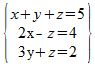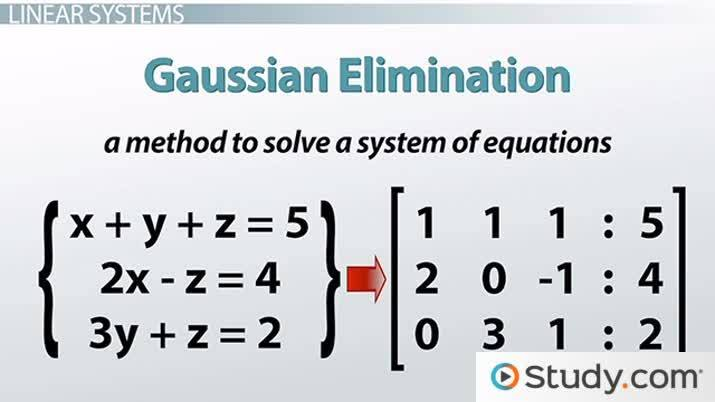An error occurred trying to load this video.

Try refreshing the page, or contact customer support.

Coming up next: How to Solve Linear Systems Using Gauss-Jordan Elimination

### You're on a roll. Keep up the good work!

Replay
Your next lesson will play in 10 seconds
• 0:01 Linear Systems
• 0:57 Augmented Matrix
• 2:16 Gaussian Elimination
• 3:57 Solving the System
• 5:20 Lesson Summary
Save Save

Want to watch this again later?

Timeline
Autoplay
Autoplay
Speed Speed

## Gaussian Elimination

A system of linear equations is a collection of two or more linear equations. Solving a system of linear equations means finding the values of all of the variables, or if that is not possible, finding the relationships between their values. In some cases, you may find that a system of linear equations has no solution. There are many ways of solving a system of linear equations. This lesson will explore just one of these methods: Gaussian elimination.

Gaussian elimination is named after Carl Friedrich Gauss, who is considered one of the most prolific mathematicians in history. In the first decade of the 18th century, he used the method to calculate the orbit of the dwarf planet Ceres by solving a system of 17 linear equations. Though it is named after Gauss (and popularized by Wilhelm Jordan), the method was actually known by the Chinese for centuries.### Creating an Augmented Matrix

In mathematics, a matrix is a rectangular array of numbers or expressions arranged into rows and columns. An example of a 2 ╳ 3 matrix is shown below.

{eq}\hspace{2em} \left[ \begin{array} {}3 & -2 & 0 \\ 1 & 1 & -7 \\ \end{array} \right] {/eq}

To use Gaussian elimination, the first step is to convert the system of linear equation into an augmented matrix, which is a matrix combined with a column on the right side, separated by a vertical line. To convert the linear equation, first move all of the terms with variables to the left side, lining like terms up vertically, and move all of the constants to the other side. The example below shows the first step.

{eq}\hspace{2em} \left\{ \begin{align*} 4x - 2 &= 5y \\[0.5em] 9x &= y \end{align*} \right. {/eq} {eq}\hspace{1em} \rightarrow \hspace{1em} {/eq} {eq}\left\{ \begin{align*} 4x - 5y &= 2 \\[0.5em] 9x - y &= 0 \end{align*} \right. {/eq}

Then use the coefficients and constants to construct an augmented matrix.

{eq}\hspace{2em} \left[ \begin{array}{cc|c} {}4 & -5 & 2 \\ 9 & -1 & 0 \\ \end{array} \right] {/eq}

An example of an augmented matrix for a system of 3 linear equation is shown below.

{eq}\hspace{2em} \left\{ \begin{align*} 2x + 5z &= 3 \\[0.5em] x - y + 5z &= 0 \\[0.5em] 4y + 3z &= -9 \end{align*} \right. {/eq} {eq}\hspace{1em} \rightarrow \hspace{1em} {/eq} {eq}\left[ \begin{array}{ccc|c} {}2 & 0 & 5 & 3 \\ 1 & -1 & 5 & 0 \\ 0 & 4 & 3 & -9 \\ \end{array} \right] {/eq}

### How to Do Gaussian Elimination?

To use Gaussian elimination, the first step is to convert the system of equations to an augmented matrix.

{eq}\hspace{2em} \left\{ \begin{align*} 2x + y - 3z &= 2 \\[0.5em] 2x - 3y - z &= 16 \\[0.5em] y + 5z &= 2 \end{align*} \right. {/eq} {eq}\hspace{1em} \rightarrow \hspace{1em} {/eq} {eq}\left[ \begin{array}{ccc|c} {}2 & 1 & -3 & 2 \\ 2 & -3 & -1 & 16 \\ 0 & 1 & 5 & 2 \\ \end{array} \right] {/eq}

The next step is to put the matrix in upper triangular form, which means making all of the values below the main diagonal equal to zero in the matrix on the left side. In the matrix above, that means that the second row must start with zero and the third row must start with two zeros. There are three basic operations that can be used to put the matrix in upper triangular form:

1. You can swap any two rows.
2. You can multiply or divide any row by a constant.
3. You can add or subtract any row from another.

For the matrix above, notice that the third row begins with a zero. Swap the second and third rows (operation 1) so that the second row begins with zero.

## Augmented Matrix

Remember that a matrix is just a rectangular array of values put into rows and columns. We first need to turn our linear system into matrix form by turning it into an augmented matrix. An augmented matrix is the combination of two matrices. In our case, we have a matrix for the coefficients of the left side of the equation and another for the right side of the equation.

Recall that turning a system of equations into matrix form involves isolating just the coefficients along with their appropriate signs after organizing them so that the x term is first followed by the y term followed by the z term, the equals sign, and then the constant. We can use a vertical line, or several dots in a vertical line, to represent our equals sign. Our linear system is already organized properly, so all we need to do is to isolate our coefficients. Our first row will have 1, 1, 1, | and then 5. Our second row has 2, 0, -1, | and 4. Our third row has 0, 3, 1, | and 2. Our matrix looks like this: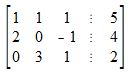## Gaussian Elimination

We can now use Gaussian elimination to help us solve this linear system. Gaussian elimination is about manipulating the augmented matrix until we have the matrix that represents the left side of the equations in upper triangular form. What this means is that we want all zeros below the main diagonal. This main diagonal starts at the top left and ends on the bottom right of the coefficients matrix. In other words, we want to manipulate the matrix so the 2 on the second row and the 0 and 3 on the third row are all 0s.

To change these numbers into 0s, we are going to use our matrix row operations. To turn our first 2 into a 0, we multiply our first row by a -2 and then we add it to the second row to create a new second row. We get a new second row of 0, -2, -3, | and -6. Now, to change the 3 in the third row into a 0, we will use this new second row combined with the third row. We will multiply the second row by 3 and add it to the third row multiplied by 2. We get a new third row of 0, 0, -7, | and -14.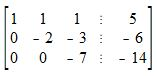To unlock this lesson you must be a Study.com Member.

Video Transcript

## Linear Systems

In math, we come across equations by themselves with just one variable that we have to solve. And then we have linear systems, a collection of linear equations. Your linear equations are equations with variables that have no exponents. So 3x + 4x = 5 is an example of a linear equation, as is x + 3y - 4z = 3.

We need one equation for each variable in our system in order to solve the system. So if we have two variables, we need two equations. If we have three variables, then we need three equations, and so on. In this video lesson, we will learn about using Gaussian elimination, a method to solve a system of equations, to help us solve our linear system. This method requires us to know how to turn our linear system into matrix form and then use simple matrix manipulations. Let's look at solving this linear system using Gaussian elimination:## Augmented Matrix

Remember that a matrix is just a rectangular array of values put into rows and columns. We first need to turn our linear system into matrix form by turning it into an augmented matrix. An augmented matrix is the combination of two matrices. In our case, we have a matrix for the coefficients of the left side of the equation and another for the right side of the equation.

Recall that turning a system of equations into matrix form involves isolating just the coefficients along with their appropriate signs after organizing them so that the x term is first followed by the y term followed by the z term, the equals sign, and then the constant. We can use a vertical line, or several dots in a vertical line, to represent our equals sign. Our linear system is already organized properly, so all we need to do is to isolate our coefficients. Our first row will have 1, 1, 1, | and then 5. Our second row has 2, 0, -1, | and 4. Our third row has 0, 3, 1, | and 2. Our matrix looks like this:## Gaussian Elimination

We can now use Gaussian elimination to help us solve this linear system. Gaussian elimination is about manipulating the augmented matrix until we have the matrix that represents the left side of the equations in upper triangular form. What this means is that we want all zeros below the main diagonal. This main diagonal starts at the top left and ends on the bottom right of the coefficients matrix. In other words, we want to manipulate the matrix so the 2 on the second row and the 0 and 3 on the third row are all 0s.

To change these numbers into 0s, we are going to use our matrix row operations. To turn our first 2 into a 0, we multiply our first row by a -2 and then we add it to the second row to create a new second row. We get a new second row of 0, -2, -3, | and -6. Now, to change the 3 in the third row into a 0, we will use this new second row combined with the third row. We will multiply the second row by 3 and add it to the third row multiplied by 2. We get a new third row of 0, 0, -7, | and -14.To unlock this lesson you must be a Study.com Member.

#### Is Gaussian elimination useful?

Gaussian elimination is a very useful method that can be used to solve a system of linear equations for its variables.

#### How does Gaussian elimination work?

There are three steps involved in Gaussian elimination:

1) Convert the system of equations to an augmented matrix.

2) Put the matrix in upper triangular form.

3) Solve for the variables starting with the last row and working your way up.

### Register to view this lesson

Are you a student or a teacher?